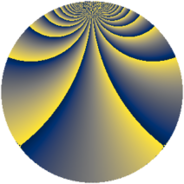# Properties

 Label 1344.4.yLevel $1344$ Weight $4$ Character orbit 1344.y Rep. character $\chi_{1344}(209,\cdot)$ Character field $\Q(\zeta_{4})$ Dimension $376$ Sturm bound $1024$

# Related objects

## Defining parameters

 Level: $$N$$ $$=$$ $$1344 = 2^{6} \cdot 3 \cdot 7$$ Weight: $$k$$ $$=$$ $$4$$ Character orbit: $$[\chi]$$ $$=$$ 1344.y (of order $$4$$ and degree $$2$$) Character conductor: $$\operatorname{cond}(\chi)$$ $$=$$ $$336$$ Character field: $$\Q(i)$$ Sturm bound: $$1024$$

## Dimensions

The following table gives the dimensions of various subspaces of $$M_{4}(1344, [\chi])$$.

Total New Old
Modular forms 1568 392 1176
Cusp forms 1504 376 1128
Eisenstein series 64 16 48

## Trace form

 $$376q + O(q^{10})$$ $$376q + 8q^{15} - 56q^{21} - 8q^{37} + 8q^{43} - 8q^{49} - 1288q^{51} - 1368q^{63} + 1640q^{67} + 16q^{79} - 8q^{81} - 1008q^{85} + 3176q^{91} + 104q^{93} + 2920q^{99} + O(q^{100})$$

## Decomposition of $$S_{4}^{\mathrm{new}}(1344, [\chi])$$ into newform subspaces

The newforms in this space have not yet been added to the LMFDB.

## Decomposition of $$S_{4}^{\mathrm{old}}(1344, [\chi])$$ into lower level spaces

$$S_{4}^{\mathrm{old}}(1344, [\chi]) \cong$$ $$S_{4}^{\mathrm{new}}(336, [\chi])$$$$^{\oplus 3}$$